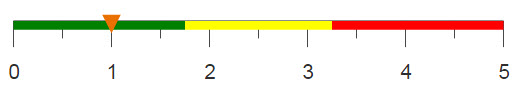# Removing values n standard deviations from the mean

You can determine outliers by identifying values in specific columns that fall n standard deviations outside of the mean for that column in a given data set. Rows that fall outside the desired range can then be eliminated.

## Difficulty## Objective

You are working with a table that contains outlier values which could skew your analysis. You want to identify all of the values that fall outside n standard deviations from the mean and eliminate them from your data set to get a more accurate picture.

## Solution

```<base table="pub.demo.weather.wunderground.observed_hourly"/>
<willbe name="avg_tempi" value="g_avg(zipcode;;tempi)"/>
<willbe name="std_tempi" value="g_std(zipcode;;tempi)"/>
<willbe name="lower_limit" value="avg_tempi-(1*std_tempi)"/>
<willbe name="upper_limit" value="avg_tempi+(1*std_tempi)"/>
<sel value="between(tempi;lower_limit;upper_limit)"/>```

## Discussion

Having outlier values in your data set can cause your analyses and aggregations to be skewed. Creating a range of acceptable values to select on will help improve results. This recipe selects values lying within one standard deviation of the mean, but you can change this number to create a different selection range.

This solution uses two g_functions: one to determine the average of the values in the data, g_avg(G;S;X), and one to determine the standard deviation, g_std(G;S;X). Upper and lower limits are created by adding and subtracting n (in this case 1) multiplied by the standard deviation from the average temperature. Then, to eliminate the values further than one standard deviation away, only the values within this range are selected.

## Further reading

If you would like to learn more about the functions and operations discussed in this recipe, click on the links below:

g_avg(G;S;X)

g_std(G;S;X)

between(X;Y;Z)